International Tables for Crystallography (2006). Vol. B, ch. 1.5, pp. 162-188   | 1 | 2 | https://doi.org/10.1107/97809553602060000553

Contents

• 1.5. Crystallographic viewpoints in the classification of space-group representations  (pp. 162-188)
• 1.5.1. List of symbols  (p. 162) | html | pdf |
• 1.5.2. Introduction  (p. 162) | html | pdf |
• 1.5.3. Basic concepts  (pp. 162-165) | html | pdf |
• 1.5.3.1. Representations of finite groups  (pp. 162-163) | html | pdf |
• 1.5.3.2. Space groups  (pp. 163-164) | html | pdf |
• 1.5.3.3. Representations of the translation groupand the reciprocal lattice  (pp. 164-165) | html | pdf |
• 1.5.3.4. Irreducible representations of space groups and the reciprocal-space group  (p. 165) | html | pdf |
• 1.5.4. Conventions in the classification of space-group irreps  (pp. 165-168) | html | pdf |
• 1.5.4.1. Fundamental regions  (pp. 165-166) | html | pdf |
• 1.5.4.2. Minimal domains  (pp. 166-167) | html | pdf |
• 1.5.4.3. Wintgen positions  (pp. 167-168) | html | pdf |
• 1.5.5. Examples and conclusions  (pp. 168-176) | html | pdf |
• 1.5.5.1. Examples  (p. 168) | html | pdf |
• 1.5.5.2. Results  (pp. 169-171) | html | pdf |
• 1.5.5.3. Parameter ranges  (pp. 171-172) | html | pdf |
• 1.5.5.4. Conclusions  (pp. 172-176) | html | pdf |
• Appendix 1.5.1. Reciprocal-space groups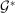(p. 176) | html | pdf |
• References | html | pdf |
• Figures
• Fig. 1.5.5.1. Symmorphic space group(p. 169) | html | pdf |
• Fig. 1.5.5.2. Symmorphic space group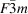(p. 171) | html | pdf |
• Fig. 1.5.5.3. ( a ), ( b ) Symmorphic space group(p. 173) | html | pdf |
• Fig. 1.5.5.4. Symmorphic space group Imm 2  (p. 177) | html | pdf |
• Tables
• Table 1.5.4.1. Conventional coefficients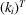of k expressed by the adjusted coefficients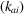of IT A for the different Bravais types of lattices in direct space  (p. 167) | html | pdf |
• Table 1.5.4.2. Primitive coefficientsof k from CDML expressed by the adjusted coefficientsof IT A for the different Bravais types of lattices in direct space  (p. 167) | html | pdf |
• Table 1.5.5.1. The k -vector types for the space groups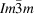and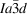(p. 168) | html | pdf |
• Table 1.5.5.2. The k -vector types for the space groupsand(p. 170) | html | pdf |
• Table 1.5.5.3. The k -vector types for the space groups,,and(p. 172) | html | pdf |
• Table 1.5.5.4. The k -vector types for the space groups Fmm 2 and Fdd 2  (pp. 174-175) | html | pdf |